# 04 MNIST Example with DataLoaders and Convolutions¶

In this notebook we will look at how data loaders can be used in pycox. This is particularly useful when working with larger data sets than what is possible to fit in memory, and is an important part of any deep learning framework. As pycox is build on torchtuples, the same principles applies as for torchtuples.Model.

For our example, we will consider the simulation study proposed by Gensheimer and Narasimhan based on the MNIST data set of handwritten digits. The basic ideas is that each digit represents a survival function, so if we can identify the digit, it is quite straight forward to get good survival estimates. We will use the LogisticHazard methods (which Gensheimer and Narasimhan refer to as Nnet-survival), with a convolutional network.

We will however, consider a slightly different survival function than that of Gensheimer and Narasimhan, and we will consider all the digits from 0 to 9, while Gensheimer and Narasimhan only considered the first 5.

In :
import numpy as np
import matplotlib.pyplot as plt

import torch
import torch.nn as nn
import torch.nn.functional as F

# MNIST is part of torchvision
from torchvision import datasets, transforms

import torchtuples as tt
from pycox.models import LogisticHazard
from pycox.utils import kaplan_meier
from pycox.evaluation import EvalSurv

In :
# for reproducability
np.random.seed(1234)
_ = torch.manual_seed(1234)


# The Dataset¶

We start by obtaining the MNIST data set with standard preprocessing. The transform ensures the data is a torch.Tensor and normalize with with a mean and standard deviation.

In :
transform = transforms.Compose(
[transforms.ToTensor(),
transforms.Normalize((0.1307,), (0.3081,))]
)
transform=transform)
mnist_test = datasets.MNIST('.', train=False, transform=transform)

In :
_ = plt.imshow(mnist_train.numpy(), cmap='gray')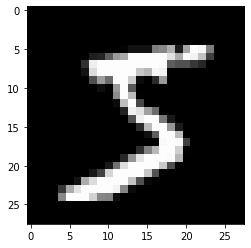## Simulation¶

Next we need to simulate the responses corresponding to the images. We draw event times from an exponential distribution with the digit defining the scale parameter

$$\beta(\text{digit}) = \frac{365 \cdot \exp(-0.6 \cdot \text{digit})}{\log(1.2)},$$

and we censor all times higher than 700.

In :
def sim_event_times(mnist, max_time=700):
digits = mnist.targets.numpy()
betas = 365 * np.exp(-0.6 * digits) / np.log(1.2)
event_times = np.random.exponential(betas)
censored = event_times > max_time
event_times[censored] = max_time
return tt.tuplefy(event_times, ~censored)


We simulate a training set and test set, based on the respective MNIST data sets.

In :
sim_train = sim_event_times(mnist_train)
sim_test = sim_event_times(mnist_test)

In :
sim_train

Out:
(array([ 21.19004682, 700.        , 104.56743096, ..., 121.80432849,
2.50843078,  13.8114342 ]),
array([ True, False,  True, ...,  True,  True,  True]))

### Visualize¶

We can visualize the survival curves for the 10 digits by applying the Kaplan-Meier estimator to the collection of event times for each digit

In :
for i in range(10):
idx = mnist_train.targets.numpy() == i
kaplan_meier(*sim_train.iloc[idx]).rename(i).plot()
_ = plt.legend()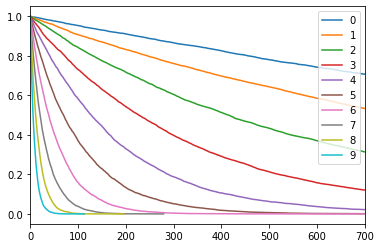Our goal will be to estimate these survival functions from the images.

## Label transforms¶

Our simulated event times are drawn in continuous time, so to apply the LogisticHazard method, we need to discretize the observations. This can be done with the label_transform attribute, and we here use an equidistant grid with 20 grid points.

In :
labtrans = LogisticHazard.label_transform(20)
target_train = labtrans.fit_transform(*sim_train)
target_test = labtrans.transform(*sim_test)


The disretization grid is

In :
labtrans.cuts

Out:
array([  0.        ,  36.84210526,  73.68421053, 110.52631579,
147.36842105, 184.21052632, 221.05263158, 257.89473684,
294.73684211, 331.57894737, 368.42105263, 405.26315789,
442.10526316, 478.94736842, 515.78947368, 552.63157895,
589.47368421, 626.31578947, 663.15789474, 700.        ])

and the discrete targets are

In :
target_train

Out:
(array([ 1, 19,  3, ...,  4,  1,  1]),
array([1., 0., 1., ..., 1., 1., 1.], dtype=float32))

To make a DataLoader we first need to create a Dataset. The DataSet is responsible for the obtaining and transforming the data, while the DataLoader is contains a DataSet a batch sampler etc.

The standard way to create a Dataset in PyTorch is by inheriting the Dataset class and defining the __getitem__ method which reads the data for one individual at a time. This also require a collate_fn for combining multiple individuals into a batch.

The following is an example of this approach, but we will shortly present an alternative approach that is more in line with torchtuples.

In :
class MnistSimDatasetSingle(Dataset):
"""Simulatied data from MNIST. Read a single entry at a time.
"""
def __init__(self, mnist_dataset, time, event):
self.mnist_dataset = mnist_dataset
self.time, self.event = tt.tuplefy(time, event).to_tensor()

def __len__(self):
return len(self.mnist_dataset)

def __getitem__(self, index):
if type(index) is not int:
raise ValueError(f"Need index to be int. Got {type(index)}.")
img = self.mnist_dataset[index]
return img, (self.time[index], self.event[index])

In :
dataset_train = MnistSimDatasetSingle(mnist_train, *target_train)
dataset_test = MnistSimDatasetSingle(mnist_test, *target_test)

In :
samp = tt.tuplefy(dataset_train)
samp.shapes()

Out:
(torch.Size([1, 28, 28]), (torch.Size([]), torch.Size([])))
In :
samp

Out:
(tensor(19), tensor(0.))

Our dataset gives a nested tuple (img, (idx_duration, event)), meaning the default collate in PyTorch does not work. We therefore use tuplefy to stack the tensors instead

In :
def collate_fn(batch):
"""Stacks the entries of a nested tuple"""
return tt.tuplefy(batch).stack()


We can now use the regular pytorch DataLoader. Note that you can set the argument num_workers in the DataLoader to use multiple processes for reading data. Dependent on the system (mac/linux/windows) this can cause some memory issues, so we here use the default num_workers = 0.

In :
batch_size = 128

In :
dl_train = DataLoader(dataset_train, batch_size, shuffle=True, collate_fn=collate_fn)
dl_test = DataLoader(dataset_test, batch_size, shuffle=False, collate_fn=collate_fn)


If we now investigate a batch, we see that we have the same tuple structure (img, (idx_durations, events)) but in a batch of size 128.

In :
batch = next(iter(dl_train))
batch.shapes()

Out:
(torch.Size([128, 1, 28, 28]), (torch.Size(), torch.Size()))
In :
batch.dtypes()

Out:
(torch.float32, (torch.int64, torch.float32))

## Dataset with batches (alternative)¶

When working with torchtuples it is typically simpler to read a batch at a times. This means that we do not need a collate_fn, and all the logic is in the Dataset. This approach is not needed, and if you prefer the regular PyTorch DataLoader, you can skip this and continue at the Convolutional Network section.

In :
class MnistSimDatasetBatch(Dataset):
def __init__(self, mnist_dataset, time, event):
self.mnist_dataset = mnist_dataset
self.time, self.event = tt.tuplefy(time, event).to_tensor()

def __len__(self):
return len(self.time)

def __getitem__(self, index):
if not hasattr(index, '__iter__'):
index = [index]
img = [self.mnist_dataset[i] for i in index]
img = torch.stack(img)
return tt.tuplefy(img, (self.time[index], self.event[index]))

In :
dataset_train = MnistSimDatasetBatch(mnist_train, *target_train)
dataset_test = MnistSimDatasetBatch(mnist_test, *target_test)

In :
samp = dataset_train[[0, 1, 3]]
samp.shapes()

Out:
(torch.Size([3, 1, 28, 28]), (torch.Size(), torch.Size()))

As we have a Dataset that reads a batch at a time, we cannot use the regular pytorch DataLoader. Instead we have to rely on the DataLoaderBatch from torchtuples, but note that we don't need the collate_fn.

In :
dl_train = tt.data.DataLoaderBatch(dataset_train, batch_size, shuffle=True)

In :
batch = next(iter(dl_train))
batch.shapes()

Out:
(torch.Size([128, 1, 28, 28]), (torch.Size(), torch.Size()))
In :
batch.dtypes()

Out:
(torch.float32, (torch.int64, torch.float32))

We see that the end result is the same as for thte DataLoader above, so use the methods you find the simplest.

# Convolutional Network¶

We will use a convolutional network with two convolutional layers, global average pooling, and two dense layers. This networks is very basic, so better performance would be expected with a more carefully designed network.

In :
class Net(nn.Module):
def __init__(self, out_features):
super().__init__()
self.conv1 = nn.Conv2d(1, 16, 5, 1)
self.max_pool = nn.MaxPool2d(2)
self.conv2 = nn.Conv2d(16, 16, 5, 1)
self.fc1 = nn.Linear(16, 16)
self.fc2 = nn.Linear(16, out_features)

def forward(self, x):
x = F.relu(self.conv1(x))
x = self.max_pool(x)
x = F.relu(self.conv2(x))
x = self.glob_avg_pool(x)
x = torch.flatten(x, 1)
x = F.relu(self.fc1(x))
x = self.fc2(x)
return x

In :
net = Net(labtrans.out_features)
net

Out:
Net(
(conv1): Conv2d(1, 16, kernel_size=(5, 5), stride=(1, 1))
(max_pool): MaxPool2d(kernel_size=2, stride=2, padding=0, dilation=1, ceil_mode=False)
(conv2): Conv2d(16, 16, kernel_size=(5, 5), stride=(1, 1))
(fc1): Linear(in_features=16, out_features=16, bias=True)
(fc2): Linear(in_features=16, out_features=20, bias=True)
)

## The Logistic-Hazard Model¶

We use the LogisticHazard with the Adam optimizer with a learning rate of 0.01.

In :
model = LogisticHazard(net, tt.optim.Adam(0.01), duration_index=labtrans.cuts)


To verify that the network works as expected we can use the batch from before

In :
pred = model.predict(batch)
pred.shape

Out:
torch.Size([128, 20])

### Training¶

We fit the network with fit_dataloader and use the dl_test to monitor the test performance. It should go without saying that, in practice, we need a validation set separate from the test set when we use early stopping, but this is just an illustrative example.

In :
callbacks = [tt.cb.EarlyStopping(patience=5)]
epochs = 50
verbose = True

0:	[33s / 33s],		train_loss: 2.0743,	val_loss: 1.9062
1:	[27s / 1m:1s],		train_loss: 1.8318,	val_loss: 1.7913
2:	[39s / 1m:40s],		train_loss: 1.7777,	val_loss: 1.7586
3:	[39s / 2m:19s],		train_loss: 1.7623,	val_loss: 1.7509
4:	[54s / 3m:14s],		train_loss: 1.7507,	val_loss: 1.7266
5:	[36s / 3m:51s],		train_loss: 1.7427,	val_loss: 1.7431
6:	[35s / 4m:27s],		train_loss: 1.7330,	val_loss: 1.7263
7:	[34s / 5m:1s],		train_loss: 1.7266,	val_loss: 1.7247
8:	[34s / 5m:36s],		train_loss: 1.7265,	val_loss: 1.7159
9:	[34s / 6m:11s],		train_loss: 1.7179,	val_loss: 1.7112
10:	[34s / 6m:46s],		train_loss: 1.7146,	val_loss: 1.7072
11:	[34s / 7m:20s],		train_loss: 1.7136,	val_loss: 1.7524
12:	[34s / 7m:55s],		train_loss: 1.7107,	val_loss: 1.7295
13:	[36s / 8m:32s],		train_loss: 1.7080,	val_loss: 1.7014
14:	[34s / 9m:6s],		train_loss: 1.7051,	val_loss: 1.7121
15:	[34s / 9m:41s],		train_loss: 1.7054,	val_loss: 1.7022
16:	[36s / 10m:18s],		train_loss: 1.7026,	val_loss: 1.7134
17:	[46s / 11m:5s],		train_loss: 1.6998,	val_loss: 1.6986
18:	[43s / 11m:48s],		train_loss: 1.7000,	val_loss: 1.7048
19:	[36s / 12m:25s],		train_loss: 1.6948,	val_loss: 1.6906
20:	[32s / 12m:58s],		train_loss: 1.6955,	val_loss: 1.6941
21:	[31s / 13m:29s],		train_loss: 1.6943,	val_loss: 1.6925
22:	[33s / 14m:2s],		train_loss: 1.6925,	val_loss: 1.6953
23:	[32s / 14m:34s],		train_loss: 1.6936,	val_loss: 1.6934
24:	[30s / 15m:5s],		train_loss: 1.6918,	val_loss: 1.6893
25:	[30s / 15m:35s],		train_loss: 1.6883,	val_loss: 1.6930
26:	[29s / 16m:5s],		train_loss: 1.6865,	val_loss: 1.6927
27:	[30s / 16m:35s],		train_loss: 1.6863,	val_loss: 1.6880
28:	[34s / 17m:10s],		train_loss: 1.6857,	val_loss: 1.6880
29:	[30s / 17m:40s],		train_loss: 1.6862,	val_loss: 1.6871
30:	[32s / 18m:13s],		train_loss: 1.6849,	val_loss: 1.6926
31:	[29s / 18m:42s],		train_loss: 1.6838,	val_loss: 1.6952
32:	[29s / 19m:12s],		train_loss: 1.6834,	val_loss: 1.6954
33:	[29s / 19m:42s],		train_loss: 1.6835,	val_loss: 1.6891
34:	[29s / 20m:11s],		train_loss: 1.6805,	val_loss: 1.6897

In :
_ = log.plot()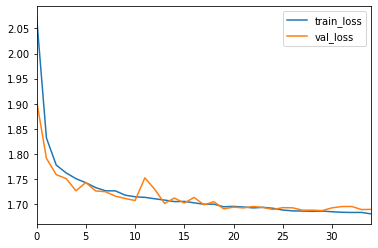# Prediction¶

To predict, we need a data loader that only gives the images and not the targets. We therefore need to create a new Dataset for this purpose.

In :
class MnistSimInput(Dataset):
def __init__(self, mnist_dataset):
self.mnist_dataset = mnist_dataset

def __len__(self):
return len(self.mnist_dataset)

def __getitem__(self, index):
img = self.mnist_dataset[index]
return img

In :
dataset_test_x = MnistSimInput(mnist_test)

In :
next(iter(dl_test_x)).shape

Out:
torch.Size([128, 1, 28, 28])

### (alternative)¶

Alternatively, if you have used the batch method, we can use the method dataloader_input_only to create this Dataloader from dl_test.

In :
dl_test_x = tt.data.dataloader_input_only(dl_test)

In :
next(iter(dl_test_x)).shape

Out:
torch.Size([128, 1, 28, 28])

### Survial predictions¶

We can obtain survival prediction in the regular manner, and one can include the interpolation if wanted.

In :
surv = model.predict_surv_df(dl_test_x)


# Results¶

We compute the average survival predictions for each digit in the test set

In :
for i in range(10):
idx = mnist_test.targets.numpy() == i
surv.loc[:, idx].mean(axis=1).rename(i).plot()
_ = plt.legend()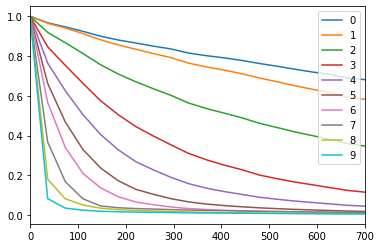and find that they are quite similar to the Kaplan-Meier estimates!

In :
for i in range(10):
idx = mnist_test.targets.numpy() == i
kaplan_meier(*sim_test.iloc[idx]).rename(i).plot()
_ = plt.legend()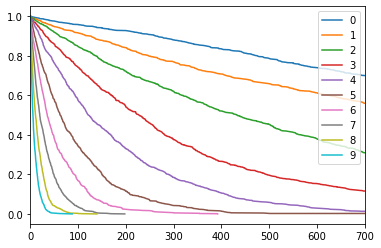### Concordance and Brier score¶

In :
surv = model.interpolate(10).predict_surv_df(dl_test_x)

In :
ev = EvalSurv(surv, *sim_test, 'km')

In :
ev.concordance_td()

Out:
0.7426348804216191
In :
time_grid = np.linspace(0, sim_test.max())
ev.integrated_brier_score(time_grid)

Out:
0.10559285952465855

# Next¶

You can now look at other examples of survival methods in the examples folder. Or, alternatively take a look at

In [ ]: